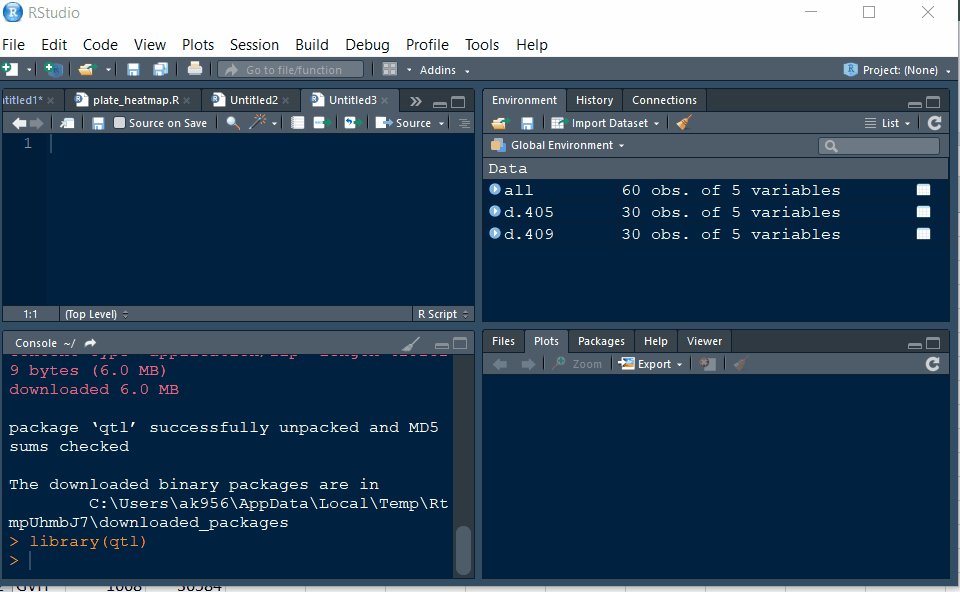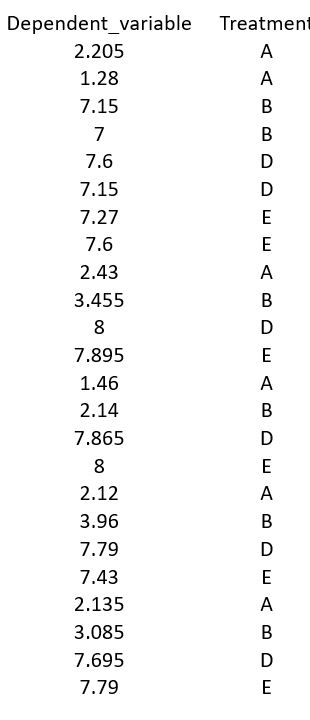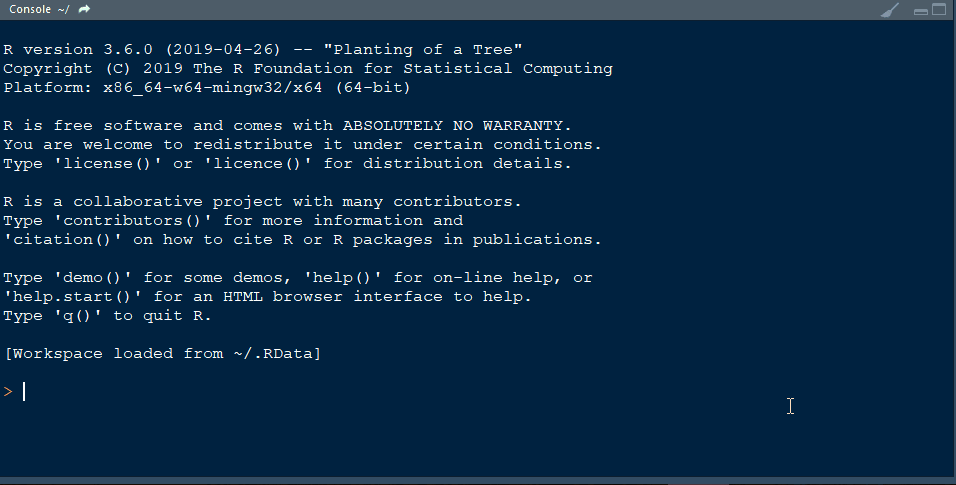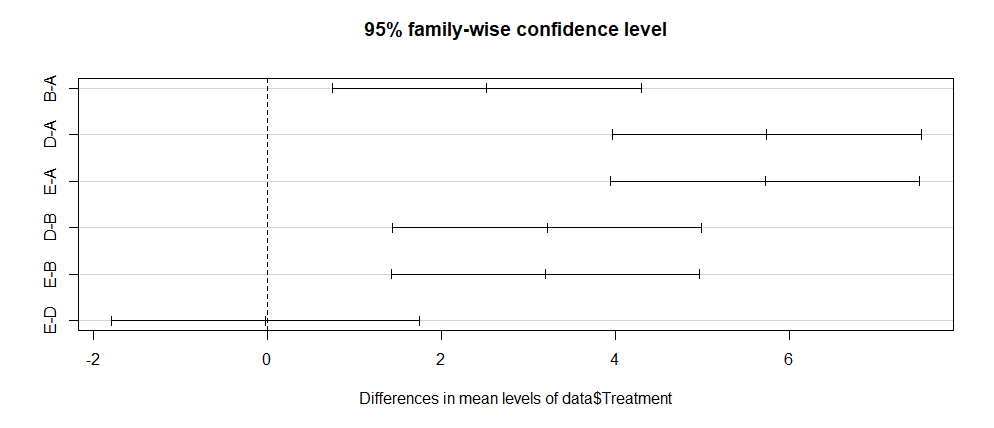ANOVA also known as Analysis of Variance is a powerful statistical method to test a hypothesis involving more than two groups (also known as treatments). However, ANOVA is limited in providing a detailed insights between different treatments or groups, and this is where, Tukey (T) test also known as T-test comes in to play. In this tutorial, I will show how to prepare input files and run ANOVA and Tukey test in R software. For detailed information on ANOVA and R, please read this article at this link.

3. Finally, install the library qtl in R

## Step 1.2 - Setup working directory following the below steps:# Step 1.3: Preparing the Input file

Create an input file as shown in the example below:# Step 2: Run ANOVA in R

### 2.1 Import R package

Install R package agricolae and open the library typing the below command line:

library(agricolae)Note: Please remember to install the correct R package for ANOVA!

### 2.2 Import data

Import your data by typing the below command line:

data= read.table(file = "fileName.txt", header = T)


### 2.3 Check data

Once the data is imported, check it by typing the below command line:

head(data_pressure)
tail(data_pressure)


### 2.4 Conduct ANOVA

Now, Simply run ANOVA by typing the below command lines:

data.lm <- lm(data$Dependent_variable ~ data$Treatment, data = data)

data.av <- aov(data.lm)
summary(data.av)


### 2.5 Regression Coefficient

Obtain regression coefficient of the predictors in the data using below code:

summary(data.lm)


Output –>

> summary(data.lm)

Call:
lm(formula = data$Dependent_variable ~ data$Treatment, data = data)

Residuals:
Min       1Q   Median       3Q      Max
-2.32500 -0.48500  0.05917  0.23979  2.68500

Coefficients:
Estimate Std. Error t value Pr(>|t|)
(Intercept)       1.9383     0.4481   4.325 0.000329 ***
data$TreatmentB 2.5267 0.6338 3.987 0.000726 *** data$TreatmentD   5.7450     0.6338   9.065 1.61e-08 ***
data$TreatmentE 5.7258 0.6338 9.035 1.70e-08 *** --- Signif. codes: 0 ‘***’ 0.001 ‘**’ 0.01 ‘*’ 0.05 ‘.’ 0.1 ‘ ’ 1 Residual standard error: 1.098 on 20 degrees of freedom Multiple R-squared: 0.8524, Adjusted R-squared: 0.8302 F-statistic: 38.49 on 3 and 20 DF, p-value: 1.692e-08  To add another coefficient, add the symbol “+” for every additional variable you want to add to the model. ### 2.6 Overall model’s performance The overall model’s performane cand be obtained using the below code: summary(data.av)  Output –> > summary(data.av) Df Sum Sq Mean Sq F value Pr(>F) data$Treatment  3  139.2   46.38   38.49 1.69e-08 ***
Residuals      20   24.1    1.20
---
Signif. codes:  0 ‘***’ 0.001 ‘**’ 0.01 ‘*’ 0.05 ‘.’ 0.1 ‘ ’ 1


### 2.7 Good fit of the linear model

The coefficient of determination or R² is a good measure to test if the linear model has the good fit, and is measured by the proportion of the total variability explained by the regression model.

R² of a linear model can be obtained using below code:


summary(data.lm)$r.squared  Output –>  summary(data.lm)$r.squared
 0.8523754



The model can explain ~85% of the total variability, which tells us that the model fits the data very well.

### 3.0 Conduct Tukey test

From the summary output, one can interpret that there is a significant difference (i.e. P < 0.001) between the Treatments, however, we perfom Tukey’s Test to investigate the differences between all treaments using steps below.

Type below commands to run Tukey test:

data.test <- TukeyHSD(data.av)
data.test


Below is the summary of the Tukey test:

> data.test
Tukey multiple comparisons of means
95% family-wise confidence level

Fit: aov(formula = data.lm)

$data$Treatment
B-A  2.52666667  0.7527896 4.300544 0.0037260
D-A  5.74500000  3.9711229 7.518877 0.0000001
E-A  5.72583333  3.9519563 7.499710 0.0000001
D-B  3.21833333  1.4444563 4.992210 0.0003106
E-B  3.19916667  1.4252896 4.973044 0.0003326
E-D -0.01916667 -1.7930437 1.754710 0.9999897


From the above T-test, one can conclude that there is a significant difference in the most of groups, except between-groups E-D at P <0.001

Finally, one can plot the above results using the below command:

plot(data.test)


Output:### --- End of Tutorial ---

Thank you for reading this tutorial. If you have any questions or comments, please let me know in the comment section below or send me an email.

### Bibliography

Felipe de Mendiburu (2019). agricolae: Statistical Procedures for Agricultural Research. R package version 1.3-1. https://CRAN.R-project.org/package=agricolae